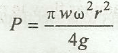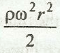# Vortex Flow

What is a vortex flow? When a cylindrical vessel, containing some liquid, is rotated about its vertical axis, the liquid surface is depressed down at the axis of its rotation and rises up near the walls of the vessel on all sides. This type of flow is known as vortex flow. It is of the following two types:

1. Forced vortex flow: In this type of flow, the vessel containing the liquid is forced to rotate about the fixed vertical axis with the help of some external torque.

2. Free vortex flow: In this type of flow, the liquid particles describe circular paths about a fixed vertical axis, without any external torque acting on the particles. The flow of water through the hole in the bottom of a wash basin is an example of free vortex flow.

The following important points may be noted for vortex flow:

(a) When a cylindrical vessel containing liquid is revolved, the surface of the liquid takes the shape of a paraboloid.

(b) The rise of liquid along the walls of a revolving cylinder about the initial level is same as the depression of the liquid at the axis of rotation.

(c) The total pressure on the bottom of a closed cylindrical vessel completely filled up with a liquid is equal to the sum of the total centrifugal pressure and the weight of the liquid in the vessel.

(d) The total pressure (P) on the top of a closed cylindrical vessel of radius (r) completely filled up with a liquid of specific weight (w) and rotating about its vertical axis is given by(e)The increase in pressure at the outer edge of a drum of radius (r) completely filled up with liquid of mass density (p) is:(f) The tangential velocity (v) of the water element having a free vortex is inversely proportional to its distance from the centre.